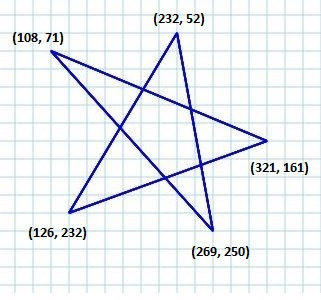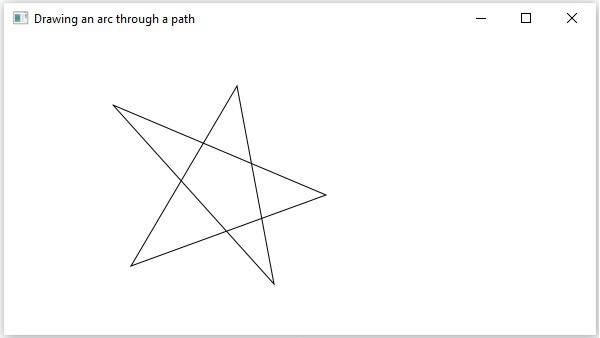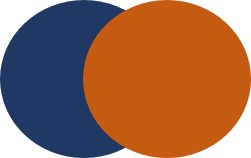# JavaFX - 2D Shapes

In the previous chapter, we have seen the basic application of JavaFX, where we learnt how to create an empty window and how to draw a line on an XY plane of JavaFX. In addition to the line, we can also draw several other 2D shapes.

## 2D Shape

In general, a 2D shape is a geometrical figure that can be drawn on the XY plane, these include Line, Rectangle, Circle, etc.

Using the JavaFX library, you can draw −

• Predefined shapes such as Line, Rectangle, Circle, Ellipse, Polygon, Polyline, Cubic Curve, Quad Curve, Arc.

• Path elements such as MoveTO Path Element, Line, Horizontal Line, Vertical Line, Cubic Curve, Quadratic Curve, Arc.

• In addition to these, you can also draw a 2D shape by parsing SVG path.

Each of the above mentioned 2D shape is represented by a class and all these classes belongs to the package javafx.scene.shape. The class named Shape is the base class of all the 2-Dimensional shapes in JavaFX.

## Creating a 2D Shape

To create a chart, you need to −

• Instantiate the respective class of the required shape.
• Set the properties of the shape.
• Add the shape object to the group.

### Instantiating the Respective Class

To create a 2 Dimensional shape, first of all you need to instantiate its respective class.

For example, if you want to create a line you need to instantiate the class named Line as follows −

```Line line = new Line();
```

### Setting the Properties of the Shape

After instantiating the class, you need to set the properties for the shape using the setter methods.

For example, to draw a line you need to pass its x and y coordinates of the start point and end point of the line. You can specify these values using their respective setter methods as follows −

```//Setting the Properties of the Line
line.setStartX(150.0f);
line.setStartY(140.0f);
line.setEndX(450.0f);
line.setEndY(140.0f);
```

### Adding the Shape Object to the Group

Finally, you need to add the object of the shape to the group by passing it as a parameter of the constructor as shown below.

```//Creating a Group object
Group root = new Group(line);
```

The following table gives you the list of various shapes (classes) provided by JavaFX.

S.No Shape & Description
1 Line

A line is a geometrical structure joining two point. The Line class of the package javafx.scene.shape represents a line in the XY plane.

2 Rectangle

In general, a rectangle is a four-sided polygon that has two pairs of parallel and concurrent sides with all interior angles as right angles. In JavaFX, a Rectangle is represented by a class named Rectangle. This class belongs to the package javafx.scene.shape.

3 Rounded Rectangle

In JavaFX, you can draw a rectangle either with sharp edges or with arched edges and The one with arched edges is known as a rounded rectangle.

4 Circle

A circle is a line forming a closed loop, every point on which is a fixed distance from a centre point. In JavaFX, a circle is represented by a class named Circle. This class belongs to the package javafx.scene.shape.

5 Ellipse

An ellipse is defined by two points, each called a focus. If any point on the ellipse is taken, the sum of the distances to the focus points is constant. The size of the ellipse is determined by the sum of these two distances.

In JavaFX, an ellipse is represented by a class named Ellipse. This class belongs to the package javafx.scene.shape.

6 Polygon

A closed shape formed by a number of coplanar line segments connected end to end. In JavaFX, a polygon is represented by a class named Polygon. This class belongs to the package javafx.scene.shape.

7 Polyline

A polyline is same a polygon except that a polyline is not closed in the end. Or, continuous line composed of one or more line segments. In JavaFX, a Polyline is represented by a class named Polygon. This class belongs to the package javafx.scene.shape.

8 Cubic Curve

A cubic curve is a Bezier parametric curve in the XY plane is a curve of degree 3. In JavaFX, a Cubic Curve is represented by a class named CubicCurve. This class belongs to the package javafx.scene.shape.

A quadratic curve is a Bezier parametric curve in the XY plane is a curve of degree 2. In JavaFX, a QuadCurve is represented by a class named QuadCurve. This class belongs to the package javafx.scene.shape.

10 Arc

An arc is part of a curve. In JavaFX, an arc is represented by a class named Arc. This class belongs to the package − javafx.scene.shape.

Types Of Arc

In addition to this we can draw three types of arc's Open, Chord, Round.

11 SVGPath

In JavaFX, we can construct images by parsing SVG paths. Such shapes are represented by the class named SVGPath. This class belongs to the package javafx.scene.shape. This class has a property named content of String datatype. This represents the SVG Path encoded string, from which the image should be drawn..

## Drawing More Shapes Through Path Class

In the previous section, we have seen how to draw some simple predefined shapes by instantiating classes and setting respective parameters.

But, just these predefined shapes are not sufficient to build complexer shapes other than the primitives provided by the javafx.shape package.

For example, if you want to draw a graphical element as shown in the following diagram, you cannot rely on those simple shapes.### The Path Class

To draw such complex structures JavaFX provides a class named Path. This class represents the geometrical outline of a shape.

It is attached to an observable list which holds various Path Elements such as moveTo, LineTo, HlineTo, VlineTo, ArcTo, QuadCurveTo, CubicCurveTo.

On instantiating, this class constructs a path based on the given path elements.

You can pass the path elements to this class while instantiating it as follows−

```Path myshape = new Path(pathElement1, pathElement2, pathElement3);
```

Or, you can get the observable list and add all the path elements using addAll() method as follows −

```Path myshape = new Path();
```

You can also add elements individually using the add() method as −

```Path myshape = new Path();
```

### The Move to Path Element

The Path Element MoveTo is used to move the current position of the path to a specified point. It is generally used to set the starting point of a shape drawn using the path elements.

It is represented by a class named LineTo of the package javafx.scene.shape. It has 2 properties of the double datatype namely −

• X − The x coordinate of the point to which a line is to be drawn from the current position.

• Y − The y coordinate of the point to which a line is to be drawn from the current position.

You can create a move to path element by instantiating the MoveTo class and passing the x, y coordinates of the new point as follows −

```MoveTo moveTo = new MoveTo(x, y);
```

If you don’t pass any values to the constructor, then the new point will be set to (0,0).

You can also set values to the x, y coordinate, using their respective setter methods as follows −

```setX(value);
setY(value);
```

## Example – Drawing a Complex Path

In this example, we will show how to draw the following shape using the Path, MoveTo and Line classes.Save this code in a file with the name ComplexShape.java.

```import javafx.application.Application;
import javafx.scene.Group;
import javafx.scene.Scene;
import javafx.stage.Stage;
import javafx.scene.shape.LineTo;
import javafx.scene.shape.MoveTo;
import javafx.scene.shape.Path;

public class ComplexShape extends Application {
@Override
public void start(Stage stage) {
//Creating a Path
Path path = new Path();

//Moving to the starting point
MoveTo moveTo = new MoveTo(108, 71);

//Creating 1st line
LineTo line1 = new LineTo(321, 161);

//Creating 2nd line
LineTo line2 = new LineTo(126,232);

//Creating 3rd line
LineTo line3 = new LineTo(232,52);

//Creating 4th line
LineTo line4 = new LineTo(269, 250);

//Creating 4th line
LineTo line5 = new LineTo(108, 71);

//Adding all the elements to the path

//Creating a Group object
Group root = new Group(path);

//Creating a scene object
Scene scene = new Scene(root, 600, 300);

//Setting title to the Stage
stage.setTitle("Drawing an arc through a path");

stage.setScene(scene);

//Displaying the contents of the stage
stage.show();
}
public static void main(String args[]){
launch(args);
}
}
```

Compile and execute the saved java file from the command prompt using the following commands.

```javac ComplexShape.java
java ComplexShape
```

On executing, the above program generates a JavaFX window displaying an arc, which is drawn from the current position to the specified point as shown below.Following are the various path elements (classes) provided by JavaFX. These classes exist in the package javafx.shape. All these classes inherit the class PathElement.

S.No Shape & Description
1 LineTo

The path element line is used to draw a straight line to a point in the specified coordinates from the current position. It is represented by a class named LineTo. This class belongs to the package javafx.scene.shape.

2 HlineTo

The path element HLineTo is used to draw a horizontal line to a point in the specified coordinates from the current position. It is represented by a class named HLineTo. This class belongs to the package javafx.scene.shape.

3 VLineTo

The path element vertical line is used to draw a vertical line to a point in the specified coordinates from the current position. It is represented by a class named VLineTo. This class belongs to the package javafx.scene.shape.

The path element quadratic curve is used to draw a quadratic curve to a point in the specified coordinates from the current position. It is represented by a class named QuadraticCurveTo. This class belongs to the package javafx.scene.shape.

5 CubicCurveTo

The path element cubic curve is used to draw a cubic curve to a point in the specified coordinates from the current position. It is represented by a class named CubicCurveTo. This class belongs to the package javafx.scene.shape.

6 ArcTo

The path element Arc is used to draw an arc to a point in the specified coordinates from the current position. It is represented by a class named ArcTo. This class belongs to the package javafx.scene.shape.

## Properties of 2D Objects

For all the 2-Dimensional objects, you can set various properties like fill, stroke, StrokeType, etc. The following section discusses various properties of 2D objects.

## Operations on 2D Objects

If we add more than one shape to a group, the first shape is overlapped by the second one as shown below.In addition to the transformations (rotate, scale, translate, etc.), transitions (animations), you can also perform three operations on 2D objects namely – Union, Subtraction and Intersection.

S.No Operation & Description
1 Union Operation

This operation takes two or more shapes as inputs and returns the area occupied by them.

2 Intersection Operation

This operation takes two or more shapes as inputs and returns the intersection area between them.

3 Subtraction Operation

This operation takes two or more shapes as an input. Then, it returns the area of the first shape excluding the area overlapped by the second one.